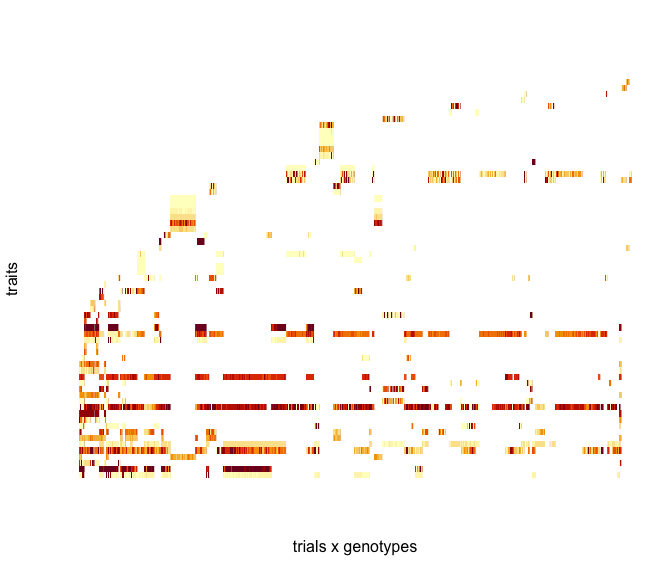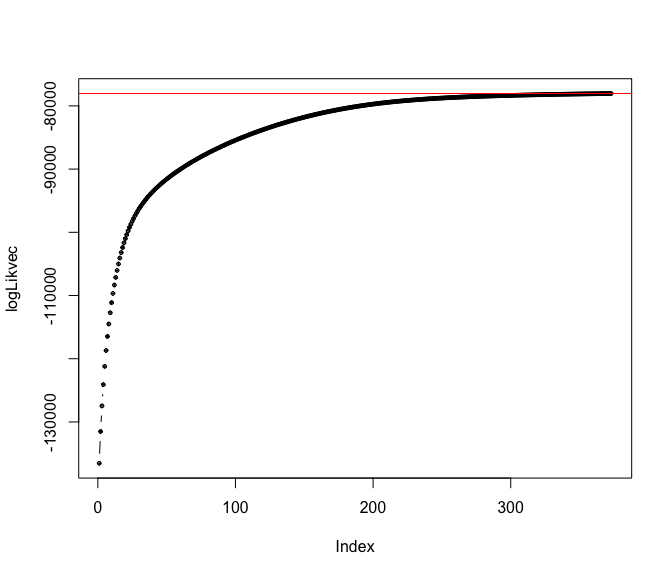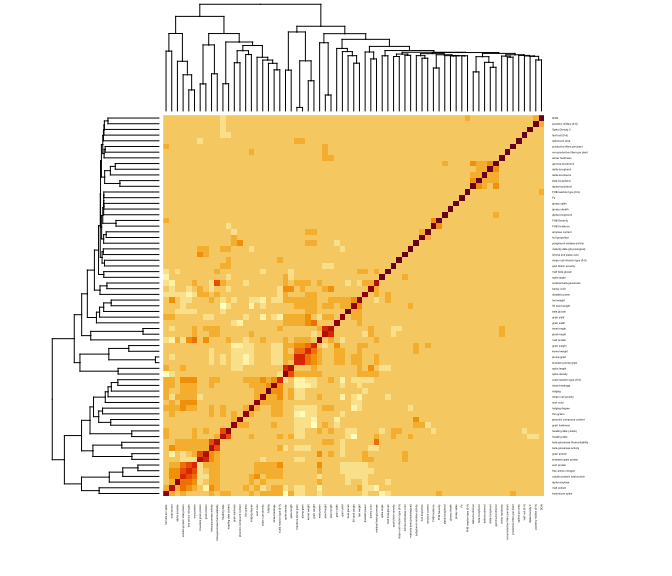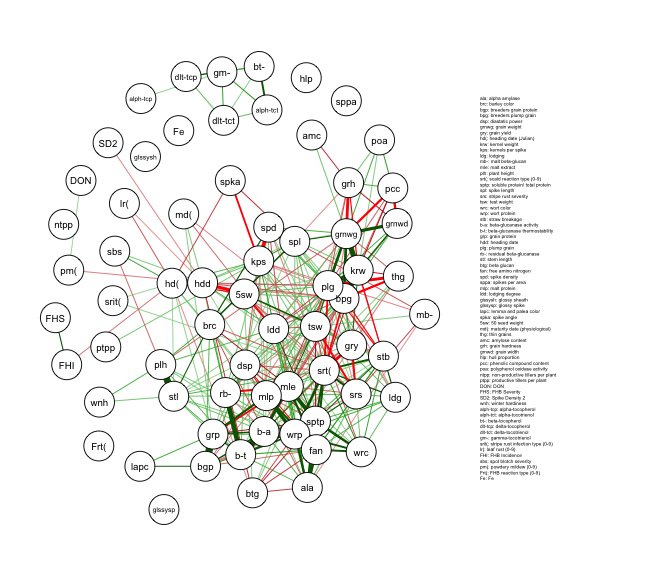# Combining partial covariance matrices using CovCombR package

#### 2020-01-13

The package is focused on combining partially overlapping relationship / covariance matrices to obtain a combined relationship / covariance matrix. At the moment the only function available to the users is the usage of which will be illustrated with an example from phenomics.

### Illustration: Combining data from independent phenotypic experimets.

Lets load the package and datasets.

library(CovCombR)
## This is CovCombR version 1.0.
## A package for combining partial covariance
## matrices from independent experiments.
## Authors: Deniz Akdemir, Julio Isidro Sanchez
## Maintainer: Deniz, <deniz.akdemir.work@gmail.com>
data("BarleyPheno")

The datasets are very heterogenous, each trial only involves a few traits. We can see this from the following image. Mean number of traits per trait is about 6.

library(plyr)
dataall<-rbind.fill(BarleyPheno)
image(as.matrix(dataall[,-c(1,2)]), xlab="trials x genotypes", ylab="traits", axes=FALSE)To use the function, we first calculate the covariance matrices from all the trials. Then we turn these into positive definite correlation matrices to likelihood convergence failures due to differences in the scaling of variables. The lis to covariance / correlation matrices become the input to the function .

covlist<-lapply(BarleyPheno, function(x){cov(x,use="pairwise.complete.obs")})
covlist<-lapply(covlist,function(x){cov2cor(as.matrix(Matrix::nearPD(x)\$mat))})
mean(c(unlist(lapply(covlist,function(x){nrow(x)}))))
##  6.113402
BigK<-CovComb(Klist=covlist, maxiter=1000,  loglik = TRUE, plotll = TRUE)Combined covariance matrix has 67 traits. Some of the relationships in this estimated relationship matrix have never been observed but wer infered by the Wishart EM algorithm.

dim(BigK[])
##  67 67

We can visualise the estimated phenomic covariance matrix.

heatmap(as.matrix(BigK[]), cexRow = .2,cexCol = .2)### Adding sparsity to the estimated covariance

Once the covariance matrix is obtained sparsity can be introduced using many of the modern sparse covariance estimation methods, for example, the packages , , , etc,…, can be used to fit sparse covariance estimators.

Graph_lasso <- qgraph::qgraph(BigK[], graph = "cor", directed=FALSE,details = FALSE,esize = 10,sampleSize=3000, layout="spring",nodeNames = rownames(BigK[]),threshold = "hochberg", legend.cex=.15)
## Registered S3 methods overwritten by 'huge':
##   method    from
##   plot.sim  BDgraph
##   print.sim BDgraph## The Wishart EM-Algorithm

In this section, we briefly describe the estimation algorithm.

Let $$A=\left\{a_1, a_2, \ldots, a_m \right\}$$ be the set of not necessarily disjoint subsets of genotypes covering a set of $$K$$ (i.e., $$K= \cup_{i=1}^m a_i$$) with total $$n$$ genotypes. Let $$G_{a_1}, G_{a_2},\ldots, G_{a_m}$$ be the corresponding sample of covariance matrices.

Starting from an initial estimate $$\Sigma^{(0)}=\nu\Psi^{(0)},$$ the Wishart EM-Algorithm repeats updating the estimate of the covariance matrix until convergence: $\begin{equation}\label{eq:covar1} \begin{split} \Psi^{(t+1)} & =\frac{1}{\nu m}\sum_{a\in A}P_a\left[ \begin{matrix} G_{aa} & G_{aa}(B^{(t)}_{b|a})' \\ B^{(t)}_{b|a}G_{aa} & \nu \Psi^{(t)}_{bb|a}+ B^{(t)}_{b|a}G_{aa}(B^{(t)}_{b|a})' \end{matrix}\right]P'_a \end{split} \end{equation}$ where $$B^{(t)}_{b|a}=\Psi^{(t)}_{ab}(\Psi^{(t)}_{aa})^{-1},$$ $$\Psi^{(t)}_{bb|a}=\Psi^{(t)}_{bb}-\Psi^{(t)}_{ab}(\Psi^{(t)}_{aa})^{-1}\Psi^{(t)}_{ba},$$ $$a$$ is the set of genotypes in the given partial covariance matrix and $$b$$ is the set difference of $$K$$ and $$a.$$ The matrices $$P_a$$ are permutation matrices that put each matrix in the sum in the same order. The initial value, $$\Sigma^{(0)}$$ is usually assumed to be an identity matrix of dimesion $$n.$$ The estimate $$\Psi^{(T)}$$ at the last iteration converts to the estimated covariance with $$\Sigma^{(T)}=\nu\Psi^{(T)}.$$

A weighted version of this algorithm can be obtained replacing $$G_{aa}$$ in Equation~ with $$G^{(w_a)}_{aa}=w_aG_{aa}+(1-w_a)\nu\Psi^{(T)}$$ for a vector of weights $$(w_1,w_2,\ldots, w_m)'.$$